# PSAT Math : How to add integers

## Example Questions

### Example Question #1 : How To Add Integers

My sister invited me to play an online word game. In the game vowels (a,e,i,o,u) are worth 3 points and consonants are worth 5. How much would I score if I use the word “University” ?

42

45

30

63

42

Explanation:

In the word we have 4 vowels (3 x 4 = 12 points) and 6 consonants (5 x 6= 30). If we add the points together we get a total of 42 points.

### Example Question #1 : How To Add Integers

What is the sum of multiples of 10 from 10 to 140 inclusive?

900
1100
1000
950
1050
Explanation:

Listing them all, 10-20-30-40-50-60-70-80-90-100-110-120-130-140 you see you can divide the numbers in half (7 pairs). Alternatively you can take (140-10+10)/2/10, adding that additional +10 in the numerator because it is inclusive, giving you 7. Just adding the top and bottom numbers gives you 10+140 for 150. 150*7 is 1050.

### Example Question #12 : Basic Operations

What number replaces the circle in the following sequence?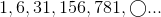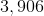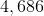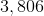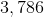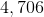Explanation:

The sequence is formed by starting with 1 and adding successive powers of 5. The numbers are obtained as follows: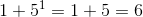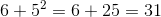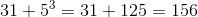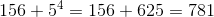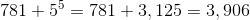- the correct response.

### Example Question #2 : How To Add Integers

Suppose you know the values of the variables in the expression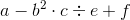and you wish to evaluate it.

Which operation do you execute last?

Squaring

Subtraction

Division

Multiplication

Explanation:

In the absence of grouping symbols, the first operations that should  be carried out are exponentiations, followed by multiplications and divisions, followed by additions and subtractions.

The additions and subtractions are carried out from left to right. Since the addition is the one to the right, it is performed last.

### Example Question #14 : Basic Operations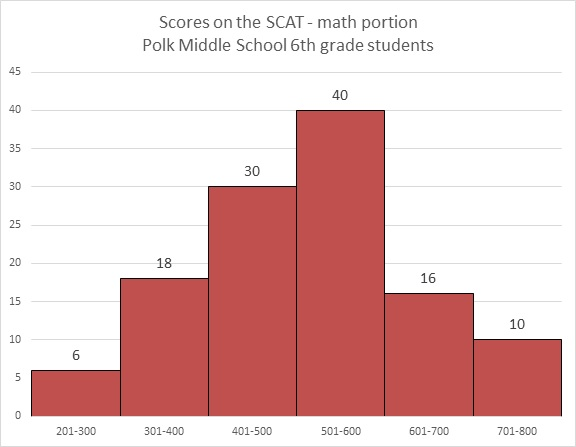Refer to the above graph. Francie, a sixth grader at Polk, scored a 372 on the math portion of the SCAT. Which of the following could have been her rank among the students?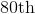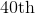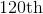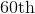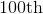Explanation:

By scoring a 372, Francie was outcored by all of the students who finished in the 401-800 ranges, which add up to: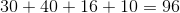students.

She was outscored by at most an additional 17 students (the other 17 in the 301-400 range), for a total of at most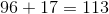students.

Francie finished between 97th and 114th place, making 100th place the only possible choice.